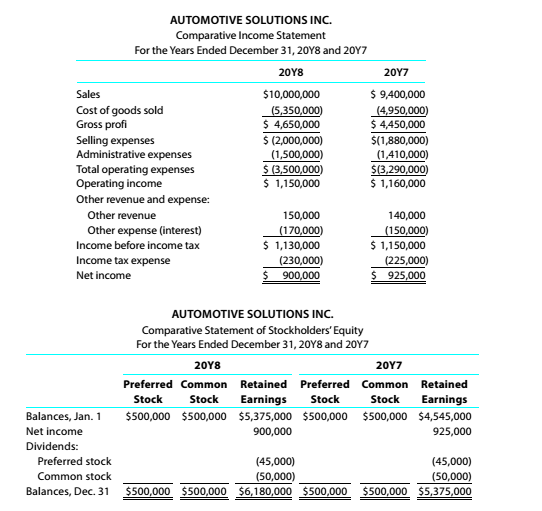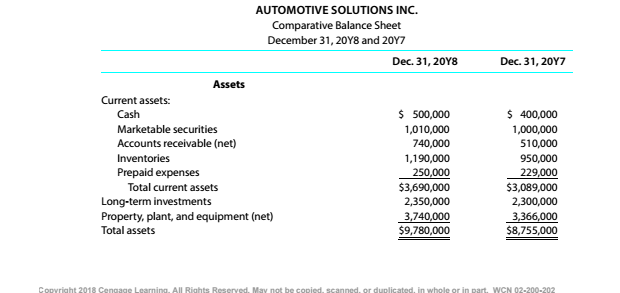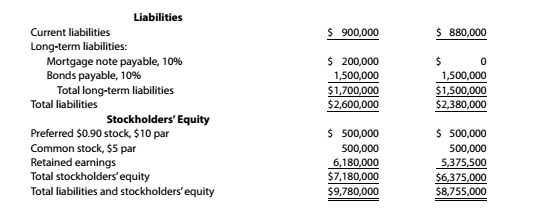Chapter 9, Problem 9.4.18P

Chapter
Section
Textbook Problem

Twenty metrics of liquidity, solvency, and profitabilityThe comparative financial statements of Automotive Solutions Inc. are as follows. The market price of Automotive Solutions Inc. common stock was $119.70 on December 31, 20Y8InstructionsPrice-earnings ration To determine Concept Introduction: Price Earnings Ratio: The price earnings ratio shows the relationship between price of the share and earnings per share. It is calculated with the help of following formula: Price Earnings Ratio=Market price per shareEarnings per share To Calculate: The Price Earnings Ratio. Explanation The Price Earnings Ratio is calculated as follows:  20Y8 Net profit (A)$ 900,000 Average Number of Common Shares outstanding (B)

Still sussing out bartleby?

Check out a sample textbook solution.

See a sample solution

The Solution to Your Study Problems

Bartleby provides explanations to thousands of textbook problems written by our experts, many with advanced degrees!

Get Started